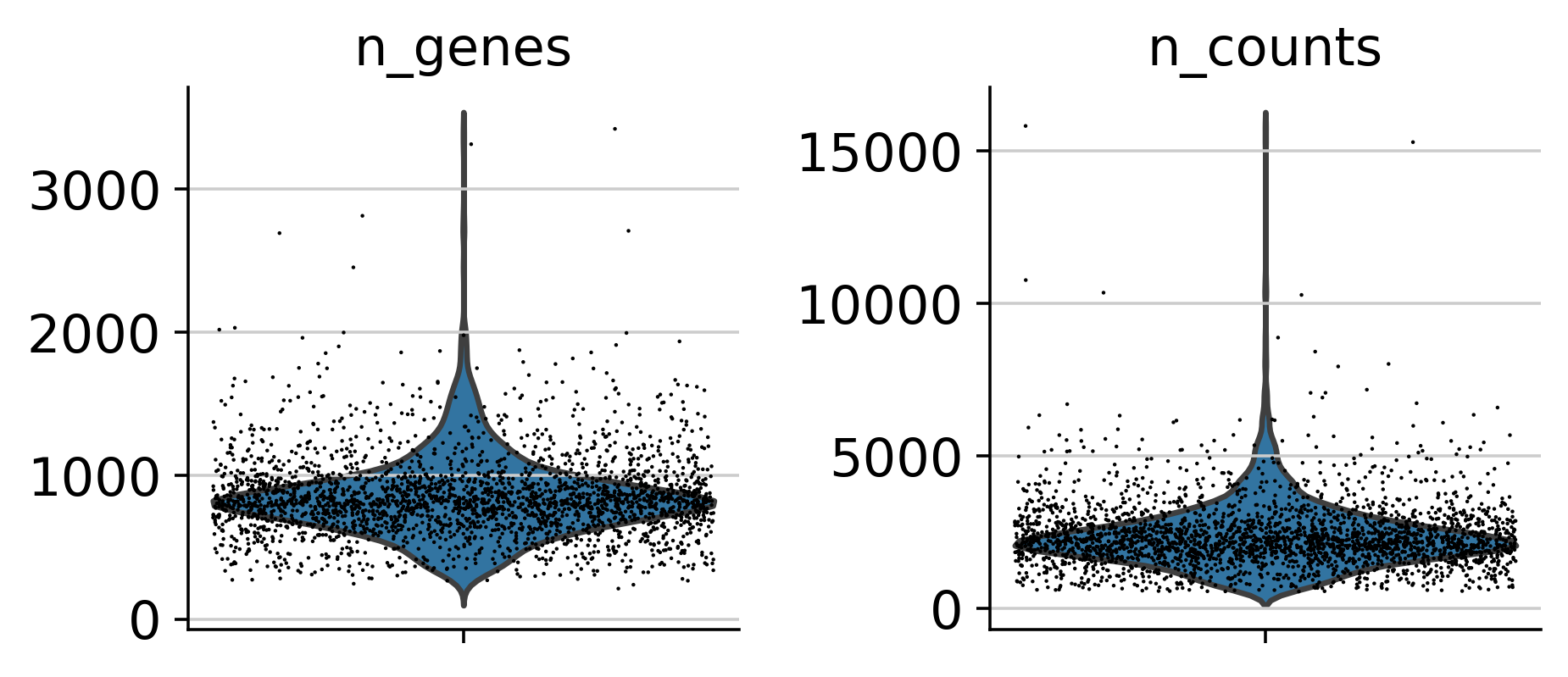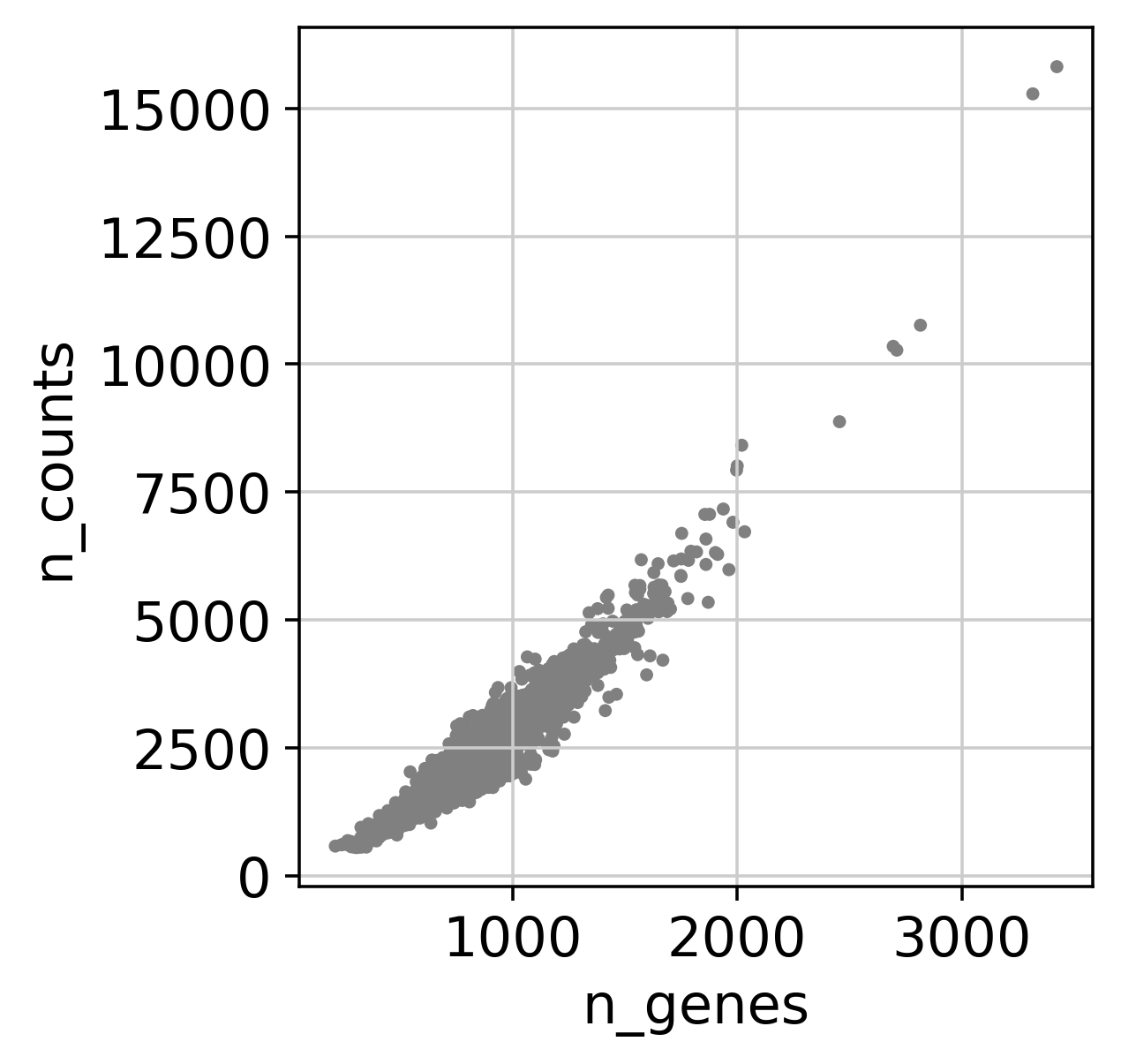In :
import numpy as np
import pandas as pd
import scanpy as sc

In :
sc.settings.verbosity = 3             # verbosity: errors (0), warnings (1), info (2), hints (3)
sc.logging.print_versions()
sc.settings.set_figure_params(dpi=160)

scanpy==1.4.4.post1 anndata==0.6.22.post1 umap==0.3.10 numpy==1.18.1 scipy==1.4.1 pandas==1.0.1 scikit-learn==0.22.2.post1 statsmodels==0.11.1 python-igraph==0.8.0 louvain==0.6.1

In :
adata = sc.read_10x_mtx(
'./filtered_gene_bc_matrices/hg19/',  # the directory with the .mtx file
var_names='gene_symbols',                  # use gene symbols for the variable names (variables-axis index)
cache=True)                                # write a cache file for faster subsequent reading

... reading from cache file cache/filtered_gene_bc_matrices-hg19-matrix.h5ad

In :
adata.var_names_make_unique()

In :
sc.pp.filter_cells(adata, min_genes=200)

filtered out 19024 genes that are detectedin less than 3 cells

In :
mito_genes = adata.var_names.str.startswith('MT-')
# for each cell compute fraction of counts in mito genes vs. all genes
# the .A1 is only necessary as X is sparse (to transform to a dense array after summing)

In :
sc.pl.violin(adata, ['n_genes', 'n_counts'], jitter=0.4, multi_panel=True)In :
sc.pl.scatter(adata, x='n_genes', y='n_counts')In :
adata = adata[adata.obs.n_genes < 2500, :]

In :
sc.pp.normalize_total(adata, target_sum=1e4)

Normalizing counts per cell.

/home/mingbo/anaconda3/envs/rs3/lib/python3.7/site-packages/scanpy/preprocessing/_simple.py:285: UserWarning: Revieved a view of an AnnData. Making a copy.
view_to_actual(data)

In :
sc.pp.highly_variable_genes(adata, min_mean=0.0125, max_mean=3, min_disp=0.5)

extracting highly variable genes
finished (0:00:00)

sc.pl.highly_variable_genes(adata)